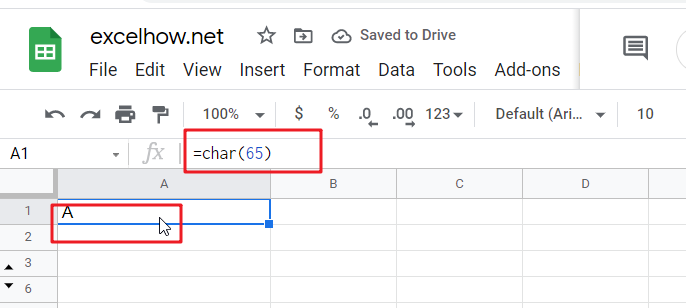# ExcelHow

This post will guide you how to use Google Sheets CODE  function with syntax and examples.

## Description

The Google Sheets CODE function returns the numeric Unicode map value for the first character of a text string. And you can get the Unicode table online at the Unicode website.

The CODE function can be used to get a numeric code for a given character or a text string. For example, if you wish to get a Unicode map value of the character “a”, and you can use the CODE(‘a’) function to get the numeric code 97 in a formula in google sheets.

The CODE function is a build-in function in Google Sheets and it is categorized as a Text Function.

## Syntax

The syntax of the CODE function is as below:

=CODE(text)

Where the CODE function argument is:

Text -This is a required argument. The text strings that you want to get the numeric Unicode map value of the first character

Note:

• You need to know that the CODE function will only convert the first character in a text string and other characters will be ignored.
• The CODE function only takes one argument, which is normally a text string or character. If the text value contains more than one character, and the CODE function only returns a Unicode map value for the first character.
• The CODE function only handle numeric input for the numbers 0-9.

## Example

#1 To get the numeric Unicode map value of the character` “c”`, just using the following CODE function:

=CODE(“c”)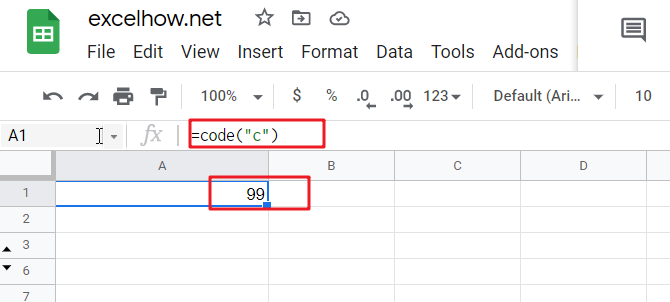#2 To get the numeric Unicode map value of the character `“c”` in cell A1, just using the following CODE function:

=CODE(A1)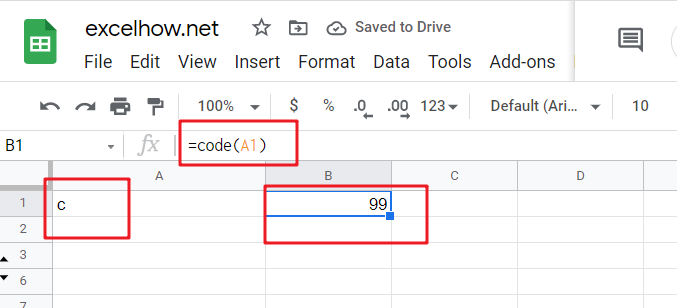#3 To get the numeric Unicode map value of string `“code”`, just using the following CODE function:

=CODE(“code”)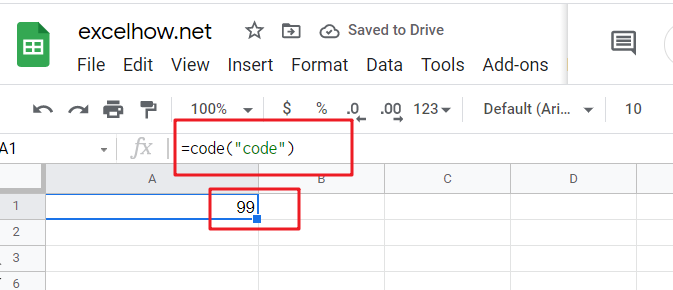#4 To get the numeric Unicode map value of numeric input for number `1`, using the following CODE function:

=CODE(1)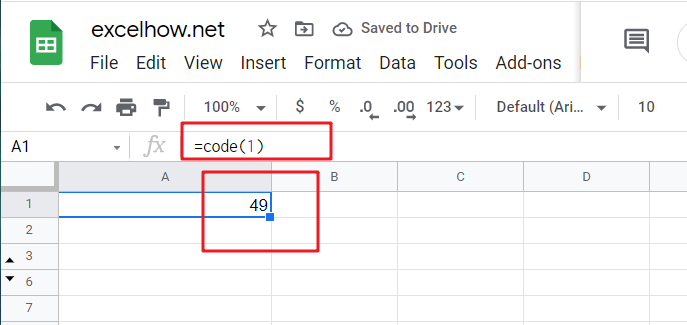So the numeric value returned by the CODE function is the code for a character in Unicode table.

If you want to get a character for given numeric code in Unicode table, and you can use the CHAR function, For example, if you want to get a Unicode character for a numeric value `65`, and you can use the following CHAR function:

=CHAR(65)